Next: Probabilistic computations for MLP Up: Standard probability distributions Previous: Normal distribution   Contents

# Dirichlet distribution

The multinomial distribution is a discrete distribution which gives the probability of choosing a given collection ofitems from a set of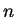items with repetitions and the probabilities of each choice given by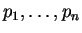. These probabilities are the parameters of the multinomial distribution .

The Dirichlet distribution is the conjugate prior of the parameters of the multinomial distribution. The probability density of the Dirichlet distribution for variables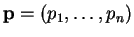with parameters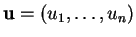is defined by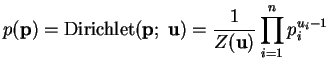(A.7)

when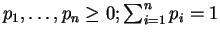and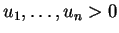. The parameterscan be interpreted as prior observation counts'' for events governed by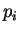. The normalisation constant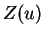becomes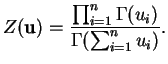(A.8)

Let. The mean and variance of the distribution are(A.9)

and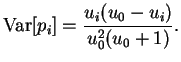(A.10)

When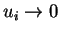, the distribution becomes noninformative. The means of all thestay the same if allare scaled with the same multiplicative constant. The variances will, however, get smaller as the parametersgrow. The pdfs of the Dirichlet distribution with certain parameter values are shown in Figure A.2.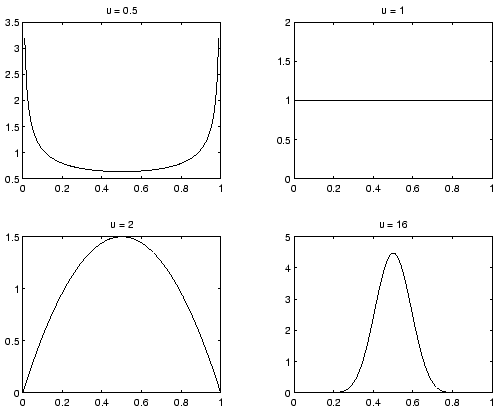In addition to the standard statistics given above, using ensemble learning for parameters with Dirichlet distribution requires the evaluation of the expectation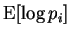and the negative differential entropy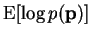.

The first expectation can be reduced to evaluating the expectation over a two dimensional Dirichlet distribution for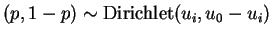(A.11)

which is given by the integral(A.12)

This can be evaluated analytically to yield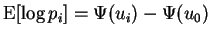(A.13)

where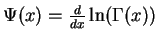is also known as the digamma function.

By using this result, the negative differential entropy can be evaluated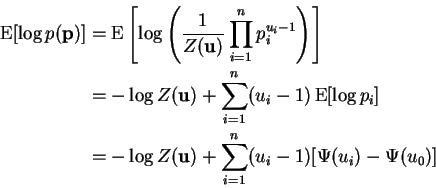(A.14)Next: Probabilistic computations for MLP Up: Standard probability distributions Previous: Normal distribution   Contents
Antti Honkela 2001-05-30